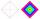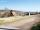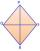Rhombus

The rhombus with area 68 has one diagonal is longer by 6 than second one.
Calculate the length of the diagonals and rhombus sides.

Result

u1 =  9.04
u2 =  15.04
a =  8.77

Solution:Leave us a comment of example and its solution (i.e. if it is still somewhat unclear...):Be the first to comment!To solve this example are needed these knowledge from mathematics:

Looking for help with calculating roots of a quadratic equation? Pythagorean theorem is the base for the right triangle calculator.

Next similar examples:

1. Diamond diagonalsCalculate the diamond's diagonal lengths if its content is 156 cm2 and the side length is 13 cm.
2. Diamond perimeterCalculate the diamond circumference which area is 288 cm square and one diagonal has a size of 124 cm.
3. DiamondDetermine the side of diamond if its content is S = 353 cm2 and one diagonal u2 = 45 cm.
4. AcreagePlot has a diamond shape, its side is 25.6 m long and the distance of the opposite sides is 22.2 meters. Calculate its acreage.
5. DiamondCalculate the length of the two diagonals of the diamond if: a = 13 cm v = 12 cm
6. Variations 4/2Determine the number of items when the count of variations of fourth class without repeating is 600 times larger than the count of variations of second class without repetition.
7. CombinationsFrom how many elements we can create 990 combinations 2nd class without repeating?
8. CombinationsHow many elements can form six times more combinations fourth class than combination of the second class?
9. EquationEquation ? has one root x1 = 8. Determine the coefficient b and the second root x2.
10. RootsDetermine the quadratic equation absolute coefficient q, that the equation has a real double root and the root x calculate: ?
11. CathetiThe hypotenuse of a right triangle is 41 and the sum of legs is 49. Calculate the length of its legs.Find the roots of the quadratic equation: 3x2-4x + (-4) = 0.Determine the discriminant of the equation: ?We want to prove the sentence: If the natural number n is divisible by six, then n is divisible by three. From what assumption we started?Which of the points belong function f:y= 2x2- 3x + 1 : A(-2, 15) B (3,10) C (1,4)Solve quadratic equation: (6n+1) (4n-1) = 3n2Given that P = (5, 8) and Q = (6, 9), find the component form and magnitude of vector PQ.